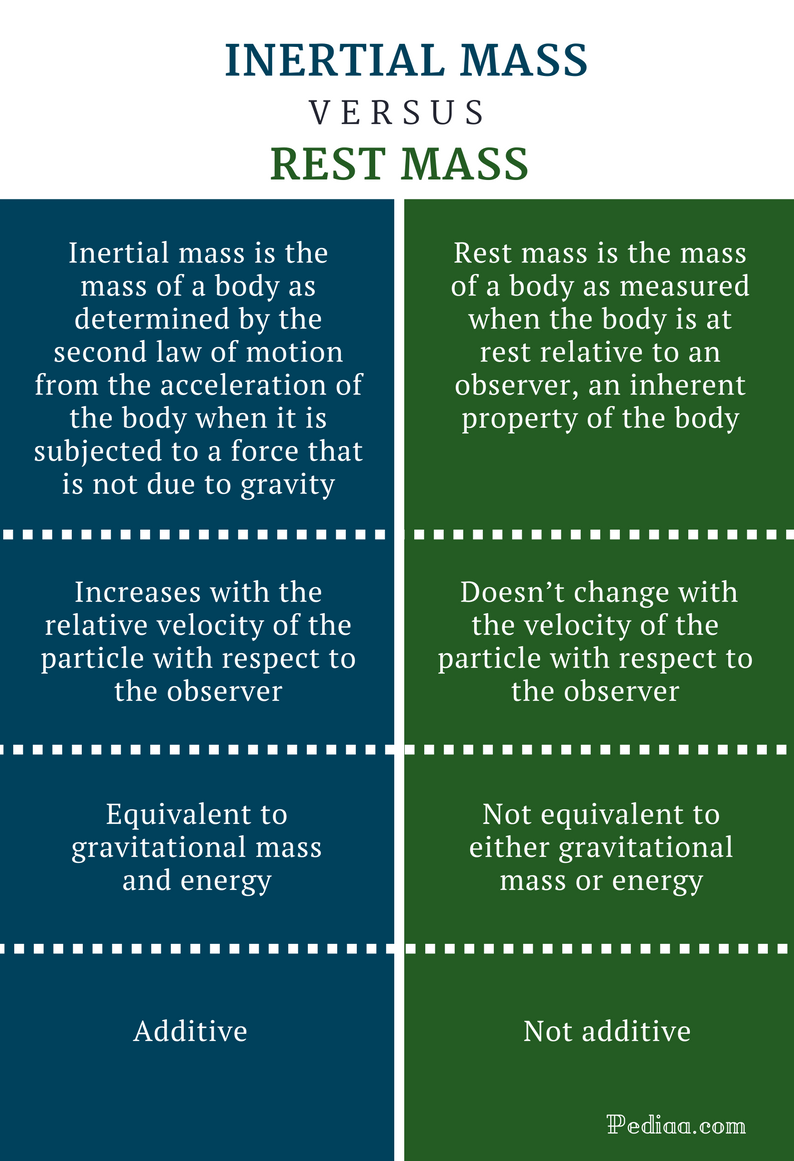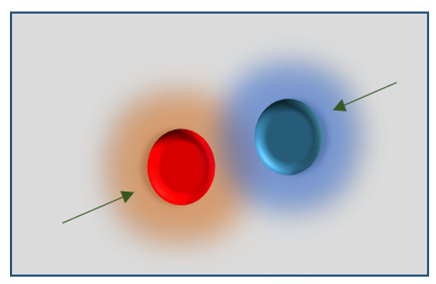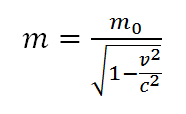# Difference Between Inertial Mass and Rest Mass

## Main Difference – Inertial Mass vs Rest Mass

Classical physics identifies only two types of masses known as inertial mass and gravitational mass. However, three types of masses are discussed in relativistic physics: rest mass, inertial mass, and gravitational mass. According to the principle of equivalence in Einstein theory of general relativity, the inertial mass and gravitational mass must be equal. The concept of rest mass is a new concept which isn’t discussed in classical physics. In modern physics, the concept of rest mass is very important, especially when we deal with low energy particles. The main difference between inertial mass and rest mass is that rest mass doesn’t change with the velocity of the particle with respect to the observer whereas inertial mass increases with the relative velocity of the particle with respect to the observer.

1. What is Inertial Mass? – Definition, Formula, Properties and Characteristics

2. What is Rest Mass? – Definition, Formula, Properties and Characteristics

3. What is the Difference between Inertial Mass and Rest Mass?## What is Inertial Mass

According to Newton’s second law of motion, the external force acting on a body is inversely proportional to its acceleration. The proportionality constant is the inertial mass of the body. Basically, if the force acting on the body is F, the inertial mass (m) of the body is given by,

m = F/a

where a, is the acceleration of the body. The inertial mass of a body is a measure of its resistance to any change in its state of motion. Higher the inertial mass, higher the force required to accelerate a given body to a given acceleration.

Sometimes, the inertial mass is also known as the relativistic mass. According to the principle of equivalence in Einstein general relativity, the inertial mass and gravitational mass are equal.## What is Rest Mass

In classical physics, the mass of an object was believed to be a constant. It was considered as an inherent property of a given object. But, in modern physics, mass isn’t an invariable. It increases with the relative velocity of the body. The mass of a moving object at a given moment is inversely proportional to the mass called the rest mass or invariant mass (also known as the proper mass) of the body. The rest mass of a given object or particle doesn’t change with its velocity. The rest mass of a given particle is the mass in its rest frame. It is an inherent property of a given particle. In general, the rest mass of a particle is denoted by m0. When the particle is moving with velocity v, with respect to the observer, the mass (m) of the particle is given by,So, the rest energy (E) of a particle having rest mass of m0 is given by E= m0c2. Where c is the speed of light through a vacuum. The rest mass of a photon is zero.

## Difference Between Inertial Mass and Rest Mass

### Definition

Rest mass: Rest mass is the mass of a body as measured when the body is at rest relative to an observer, an inherent property of the body.

Inertial mass: Inertial mass is the mass of a body as determined by the second law of motion from the acceleration of the body when it is subjected to a force that is not due to gravity.

### Nature of the Quantity

Rest mass: Rest mass is an inherent quantity of a given particle which doesn’t change with the velocity of the particle with respect to the observer.

Inertial mass: Inertial mass of a particle increases with the relative velocity of the particle with respect to the observer. The inertial mass of a given system is greater than or equal to its rest mass.

### Upper and Lower Limits

Rest mass: The rest mass of a given particle doesn’t change at all.

Inertial mass: If a given particle is at rest with respect to the observer, the inertial mass is equal to its rest mass. It is the lowest inertial mass of the particle. But, the mass of a particle reaches infinity as the velocity of the particle relative to the observer reaches the velocity of light. According to the theory of special relativity, a particle requires an infinite amount of energy to accelerate to the speed of light.

### Importance

Rest mass: If a given particle is moving at a low speed relative to the observer, the mass of the particle is very close to its rest mass. But at high speeds, the rest mass remains unchanged while the mass of the particle increases dramatically with speed.

Inertial mass: At very high speeds, the mass of a given particle is much greater than its rest mass.

Rest mass: Rest mass is not additive.

Inertial mass: Inertial mass is additive.

### Equivalence

Rest mass: Rest mass is not equivalent to either gravitational mass or energy.

Inertial mass: Inertial mass is equivalent to gravitational mass and energy.